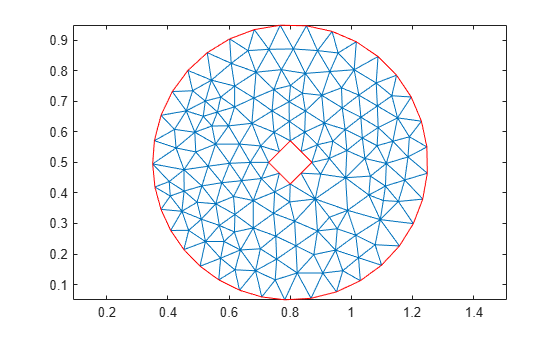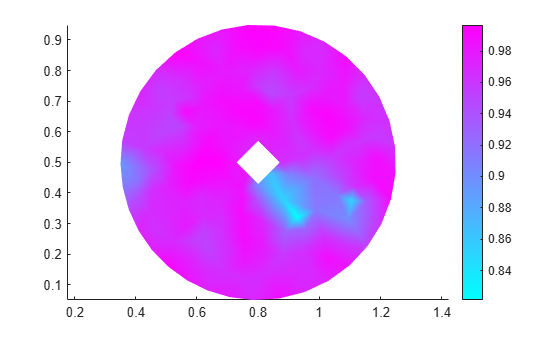# pdetriq

(Not recommended) Triangle quality measure

`pdetriq` is not recommended. Use `meshQuality` instead.

## Syntax

``q = pdetriq(p,t)``

## Description

example

````q = pdetriq(p,t)` returns a row vector of numbers from 0 through 1 representing the triangle quality of all the elements of the `[p,e,t]` mesh.`pdetriq` evaluates the quality of a triangle as$q=\frac{4a\sqrt{3}}{{h}_{1}^{2}+{h}_{2}^{2}+{h}_{3}^{2}}$where a is the area and h1, h2, and h3 are the lengths of the edges of the triangle.The value 0 corresponds to a degenerate triangle with zero area. The value 1 corresponds to a triangle with h1 = h2 = h3.```

## Examples

collapse all

Evaluate the quality for each triangle of a `[p,e,t]` mesh.

Generate and plot a mesh for the geometry consisting of a circle with a diamond hole.

```[p,e,t] = initmesh(@scatterg); pdemesh(p,e,t) axis equal```Evaluate the triangle quality for each mesh triangle. Plot the resulting quality values.

```q = pdetriq(p,t); figure pdeplot(p,e,t,'XYData',q); axis equal```## Input Arguments

collapse all

Mesh nodes, specified as a 2-by-`Np` matrix of nodes (points), where `Np` is the number of nodes in the mesh. For details on the mesh data representation, see `initmesh`.

Data Types: `double`

Mesh elements, specified as a `4`-by-`Nt` matrix of triangles, where `Nt` is the number of triangles in the mesh. For details on the mesh data representation, see `initmesh`.

Data Types: `double`

## Output Arguments

collapse all

Triangle quality, returned as a row vector of numbers from 0 through 1.

 Bank, Randolph E. PLTMG: A Software Package for Solving Elliptic Partial Differential Equations, User's Guide 6.0. Philadelphia, PA: Society for Industrial and Applied Mathematics, 1990.